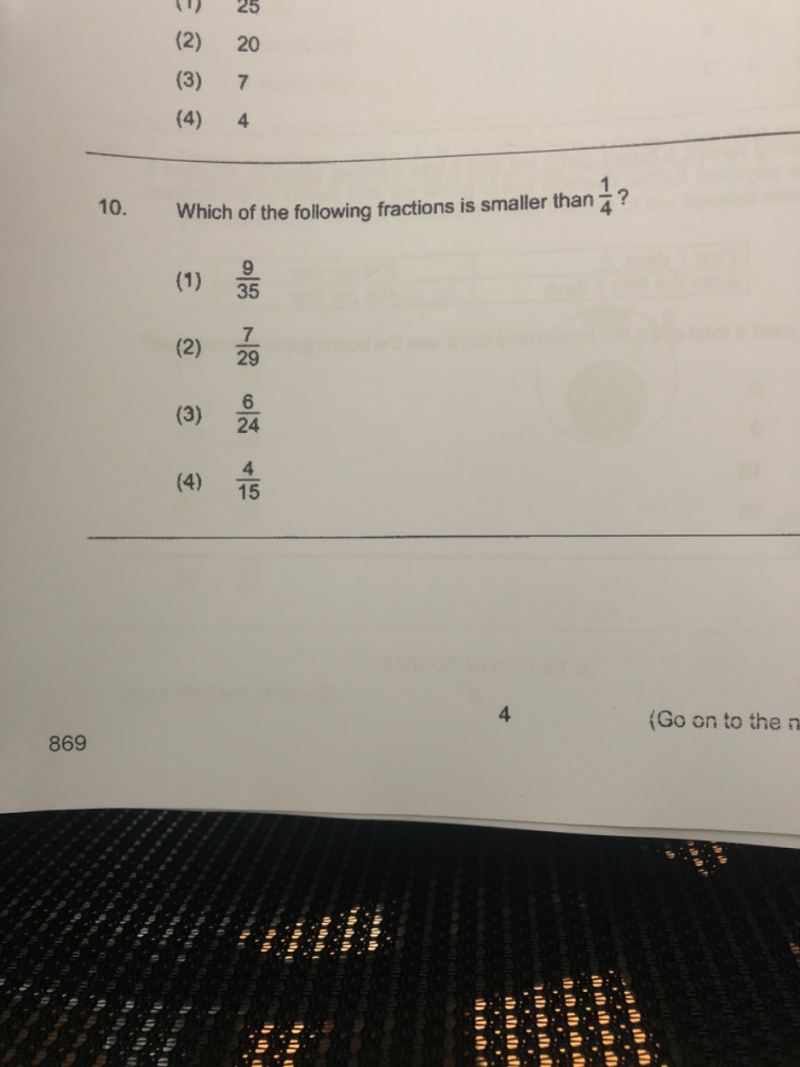# QuestionPls help. Thanks

Find the quarter point and compare with the numerator.

Quarter point          Numerator       Check

(1)   35/4       = 8.75          <             9                      ❌

(2)   29/4      = 7.25          >              7                     ✔️

(3)   24/4      =  6              =              6                     ❌

(4)   15/4       = 3.75          <             4                     ❌

Hence the answer is (2).

Please click on “Accept Answer” if the answer is correct.

0 Replies 1 Like ✔Accepted Answer

The answer is 2. Just make numerators same and the one with smaller denominator is bigger.

0 Replies 0 Likes